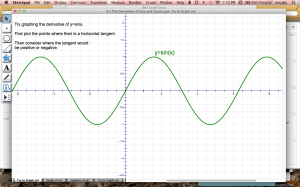# GSP and the Derivative of the Sine FunctionToday in MCV4U we will investigate the derivative of the Sine and Cosine functions. First, download theand save it to the camera roll. Open the image in the app Explain Everything. Follow the steps below.

Try graphing the derivative of y=sinx.
First plot the points where their is a horizontal tangent.
Then consider where the tangent would
be positive or negative.

Next, download the GSP file and open it in Sketchpad Explorer. On the “Graph Sin’ ” page drag the point back and forth which will plot the points corresponding to the slope of the tangent.